# GSEB Solutions Class 6 Maths Chapter 5 Understanding Elementary Shapes Ex 5.2

Gujarat Board GSEB Textbook Solutions Class 6 Maths Chapter 5 Understanding Elementary Shapes Ex 5.2 Textbook Questions and Answers.

## Gujarat Board Textbook Solutions Class 6 Maths Chapter 5 Understanding Elementary Shapes Ex 5.2

Question 1.
What fraction of a clockwise revolution does the hour hand of a clock turn through, when it goes from.
(a) 3 to 9
(b) 4 to 7
(c) 7 to 10
(d) 12 to 9
(e) 1 to 10
(f) 6 to 3
Solution:
(a) 3 to 9
The hour hand going from 3 to 9 turns through $$\frac { 1 }{ 2 }$$ of a revolution.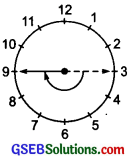(b) 4 to 7
The hour hand going from 4 to 7 turns through $$\frac { 1 }{ 4 }$$ of a revolution.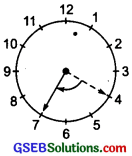(c) 7 to 10:
The hour hand going from 7 to 10 turns through $$\frac { 1 }{ 4 }$$ 3f a revolution.(c) 12 to 9:
The hour hand going from 12 to 9 turns through $$\frac { 3 }{ 4 }$$ of a revolution.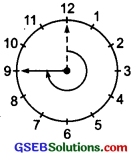(d) 1 to 10:
The hour hand going from 1 to 10 turns through $$\frac { 3 }{ 4 }$$ of a revolution.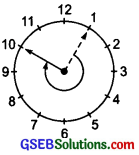(f) 6 to 3:
The hour hand going from 6 to 3 turns through of a revolution.Question 2.
Where will the hand of a clock stop if it
(a) starts at 12 and makes $$\frac { 1 }{ 2 }$$ of a revolution, clockwise?
(b) starts at 2 and makes $$\frac { 1 }{ 2 }$$ of a revolution, clockwise?
(c) starts at 5 and makes $$\frac { 1 }{ 4 }$$ of a revolution, clockwise?
(d) starts at 5 and makes $$\frac { 3 }{ 4 }$$ of a revolution, clockwise?
Solution:
The clock hand starting: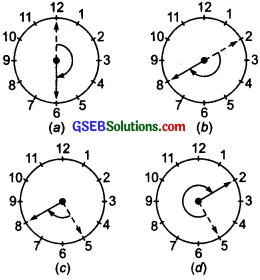(a) from 12 and making $$\frac { 1 }{ 2 }$$ of a revolution reaches at 6.
(b) from 2 and making $$\frac { 1 }{ 2 }$$ of a revolution reaches at 8.
(c) from 5 and making $$\frac { 1 }{ 4 }$$ of a revolution reaches at 8.
(d) from 5 and making $$\frac { 3 }{ 4 }$$ of a revolution reaches at 2.Question 3.
Which direction will you face f you start facing
(i) (a) east and make $$\frac { 1 }{ 2 }$$ of a revolution clockwise?
(b) east and make 1$$\frac { 1 }{ 2 }$$ of a revolution clockwise?
(c) west and make $$\frac { 3 }{ 4 }$$ of a revolution anti clockwise?
(d) south and make one full revolution?
(ii) Should we specify clockwise or anti-clockwise
Solution:
(i) From the figure, we can say that the facing will be towards:
(a) West
(b) West
(c) North
(d) South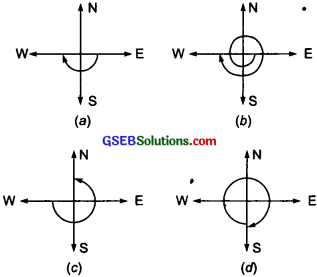Question 4.
What part of a revolution have you turned through f you stand facing
(a) east and turn clockwise to face north?
(b) south and turn clockwise to face east?
(c) west and turn clockwise to face east?
Solution:
(a) Starting from east and reaching at north (turning clockwise). $$\frac { 3 }{ 4 }$$ of a revolution is required.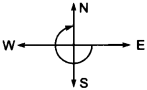(b) Starting from south and turning clockwise up to east, $$\frac { 3 }{ 4 }$$ of a revolution is required.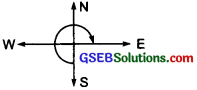(c) Starting from west and turning clockwise up to east $$\frac { 1 }{ 2 }$$ of a revolution is required.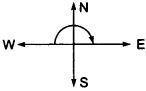Question 5.
Find the number of right angles turned through by the hour hand of a clock when it goes from
(a) 3 to 6
(b) 2 to 8
(c) 5 to 11
(d) 10 to 1
(e) 12 to 9
(f) 12 to 6
Solution:
(a) 3 to 6:
The hour hand going from 3 to 6 turns through 1 right angle.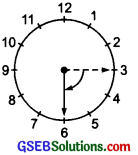(b) 2 to 8:
The hour hand going from 2 to 8 turns through 2 right angles.(c) 5 to 11:
The hour hand going from 5 to 11 turns through 2 right angles.(d) 10 to 1:
The hour hand going from 10 to 1 turns through 1 right angle.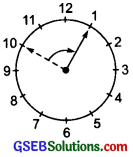(e) 12 to 9:
The hour hand going from 12 to 9 turns through 3 right angles.(f) 12 to 6:
The hour hand going from 12 to 6 turns through 2 right angles.Question 6.
How many right angles do you make if you start facing
(a) south and turn clockwise to west?
(b) north and turn anti-clockwise to east?
(c) west and turn to west?
(d) south and turn to north?
Solution:
(a) From the figure, we Observe that turning clockwise from south to west, we make 1 right angle.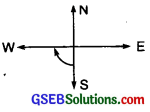(b) From the figure, we find that turning anti-clockwise from north to east, we make 3 right angles.(c) From the figure, we find that turning [clockwise or anti-clockwise] from west to west, we make 4 right angles.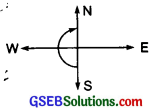(d) From the figure, we find that turning [clockwise or anti-clockwise] from south to north, we make 2 right angles.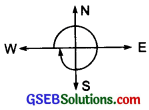Question 7.
Where will the hour hand of a clock stop if it starts
(a) from 6 and turns through 1 right angle?
(b) from 8 and turns through 2 right angles?
(c) from 10 and turns through 3 right angles?
(d) from 7 and turns through 2 straight angles?
Solution:
(a) Starting from 6 and turning through 1 right angle, the hour hand will reach at 9.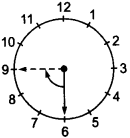(b) Starting from 8 and turning through 2 right angles, the hour hand will reach at 2.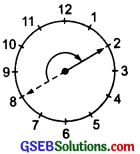(c) Starting from 10 and turning through 3 right angles, the hour hand will reach at 7.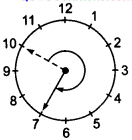(d) Starting from 7 and turning through 2 straight angles, the hour hand will reach at 7.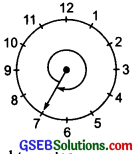Note: 2 straight angles = 4 right angles